# Interactive Math Games

Are you looking for interactive math games? Here you can find interesting math games that you can play at home or at school. Learning math can be so much fun!

The following math games are suitable for elementary and middle school students.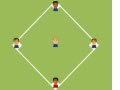Rounding Decimals Game

Practice rounding decimals when playing this fun multi-player baseball game.Monster Math - Integer Math Game
You will have so much fun catching and destroying different monsters when playing this interactive game about integers. To get to the bonus round, you must first add and subtract integers correctly.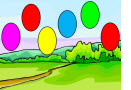Adding Integers Balloon Game

This is another fun game that rewards speed and accuracy when adding integers. Check it out!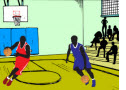Mixed Operations Basketball Game

Apply the correct order of operations in order to find the right answer and get a chance to catch and throw the ball.Multiplying Numbers Ending in Zeroes

Find the treasure chest by making no mistakes when multiplying numbers ending in zeroes.Baseball Division Game

Practice dividing by one digit numbers when playing this interactive multi-player baseball game.Concentration Game With Integers

In this concentration game, you will have to match each sum or difference with the correct integer.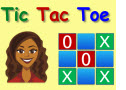Even or Odd?

Classify numbers as even or odd by playing this fun Tic-tac-Toe game against the computer.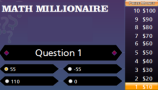Absolute Value Millionaire

In this millionaire-style game, students will find the absolute value of different numbers and expressions.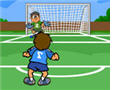Properties of Addition Soccer Game

In this interactive two-player soccer game students will identify and use different properties of addition.Short Division Basketball Game

Third grade students will have fun dividing small numbers when playing this math basketball game.

Return from the Interactive Math Games page to the Math Play homepage.